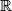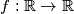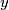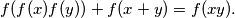### IMO Shortlist 2017 problem A6

Kvaliteta:
Avg: 0.0
Težina:
Avg: 8.0

Let$\mathbb{R}$ be the set of real numbers. Determine all functions$f: \mathbb{R} \rightarrow \mathbb{R}$ such that, for any real numbers$x$ and$y$,Source: https://www.imo-official.org/problems/IMO2017SL.pdf